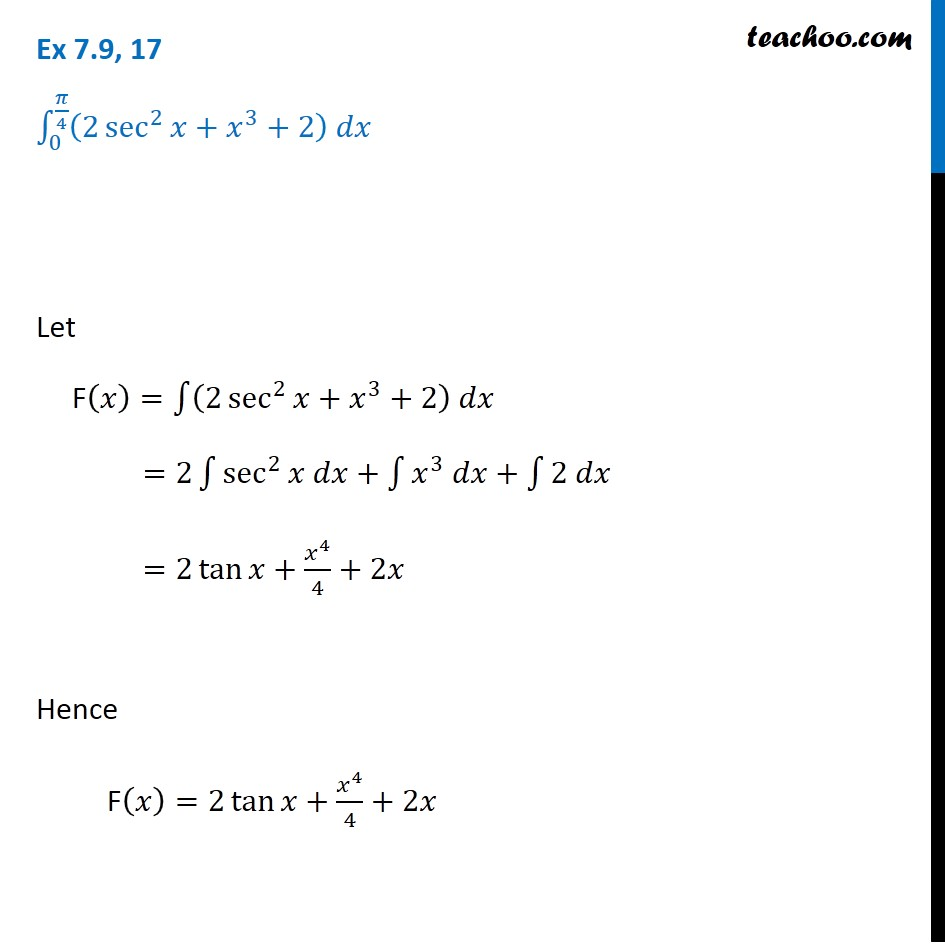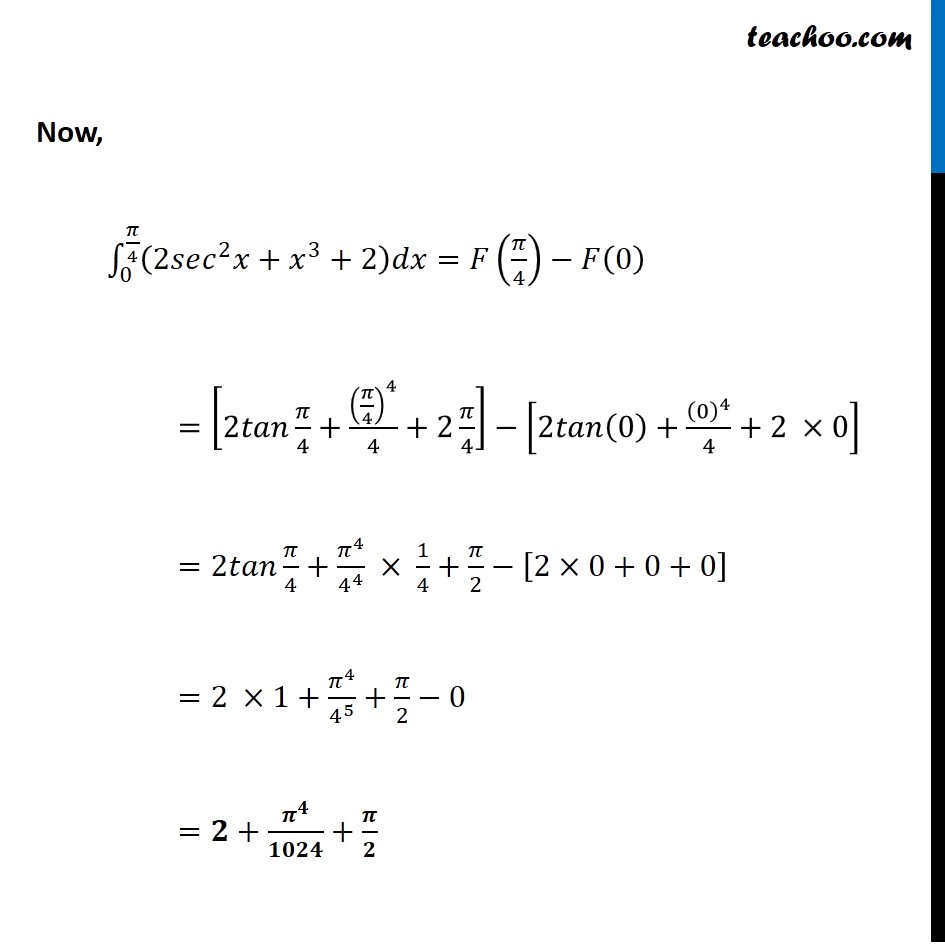Ex 7.9

Chapter 7 Class 12 Integrals
Serial order wiseGet live Maths 1-on-1 Classs - Class 6 to 12

### Transcript

Ex 7.9, 17 ∫_0^(𝜋/4)▒(2 sec^2⁡𝑥+𝑥^3+2) 𝑑𝑥 Let F(𝑥)=∫1▒〖(2 sec^2⁡〖𝑥+𝑥^3+2〗 ) 𝑑𝑥〗 =2∫1▒〖sec^2⁡〖𝑥 𝑑𝑥〗+∫1▒〖𝑥^3 𝑑𝑥+∫1▒〖2 𝑑𝑥〗〗〗 =2 tan⁡〖𝑥+𝑥^4/4+2𝑥〗 Hence F(𝑥)=2 tan⁡〖𝑥+𝑥^4/4+2𝑥〗 Now, ∫_0^(𝜋/4)▒〖(2𝑠𝑒𝑐^2 𝑥+𝑥^3+2)𝑑𝑥=𝐹(𝜋/4)−𝐹(0) 〗 =[2𝑡𝑎𝑛 𝜋/4+(𝜋/4)^4/4+2 𝜋/4]−[2𝑡𝑎𝑛(0)+(0)^4/4+2 ×0] =2𝑡𝑎𝑛 𝜋/4+𝜋^4/4^4 × 1/4+𝜋/2−[2×0+0+0] =2 ×1+𝜋^4/4^5 +𝜋/2−0 =𝟐+𝝅^𝟒/𝟏𝟎𝟐𝟒+𝝅/𝟐